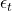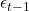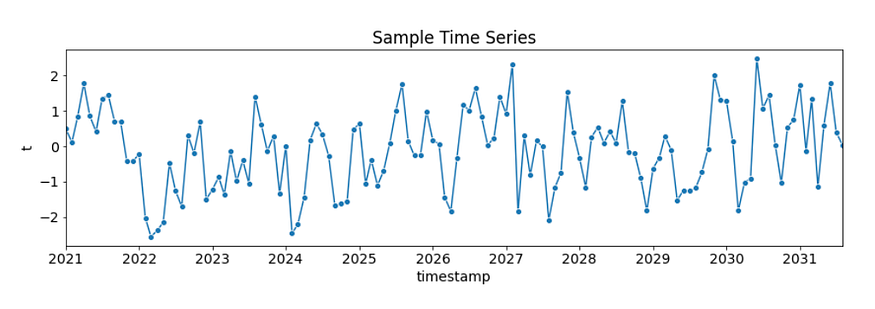2022-01-25
###### Understanding the Dummy Variable Trap with example
2022-01-27

Autocorrelation analysis is an important step in the Exploratory Data Analysis of time series forecasting. The autocorrelation analysis helps detect patterns and check for randomness. It’s especially important when you intend to use an autoregressive–moving-average (ARMA) model for forecasting because it helps to determine its parameters. The analysis involves looking at the Autocorrelation Function (ACF) and Partial Autocorrelation Function (PACF) plots. This article helps you build an intuition for interpreting ACF and PACF plots.

This article helps you build an intuition for interpreting these ACF and PACF plots. We’ll briefly go over the fundamentals of the ACF and PACF. However, as the focus lies on the interpretation of the plots, a detailed discussion of the underlying mathematics is beyond the scope of this article. We’ll refer to other resources instead. This article is a revisited version of this Kaggle Notebook, which was originally published in December 2021.

## Fundamentals

The ACF and PACF plots are used to figure out the order of AR, MA, and ARMA models. In this section, we’ll only briefly touch on the relevant terms. For detailed explanations, we’ll refer to other resources.

## Auto-Regressive and Moving Average Models

Auto-Regressive Model

The Auto-Regressive (AR) model assumes that the current value (y_t) is dependent on previous values (y_(t-1), y_(t-2), …). Because of this assumption, we can build a linear regression model.

To figure out the order of an AR model, you need to look at the PACF.

Moving Average Model

The Moving Average (MA) model assumes that the current value (y_t) is dependent on the error terms including the current error (,,…). Because error terms are random, there’s no linear relationship between the current value and the error terms.

To figure out the order of an MA model, you need to look at the ACF.

## Precondition: Stationarity

ACF and PACF assume stationarity of the underlying time series.

## Autocorrelation Function (ACF) and Partial Autocorrelation Function (PACF)

The ACF and PACF are used to figure out the order of AR, MA, and ARMA models.

Autocorrelation Function (ACF)

Autocorrelation is the correlation between a time series with a lagged version of itself. The ACF starts at a lag of 0, which is the correlation of the time series with itself and therefore results in a correlation of 1.

We’ll use the plot_acf function from the statsmodels.graphics.tsaplots library. For this article, we’ll only look at 15 lags since we are using minimal examples.

from statsmodels.graphics.tsaplots import plot_acf
plot_acf(time_series_values, lags = 15)


The ACF plot can provide answers to the following questions:

• Is the observed time series white noise/random?
• Is an observation related to an adjacent observation, an observation twice-removed, and so on?
• Can the observed time series be modeled with an MA model? If yes, what is the order?

Partial Autocorrelation Function (PACF)

The partial autocorrelation at lag k is the autocorrelation between X_t and X_(t-k) that is not accounted for by lags 1 through k−1.

We’ll use the plot_pacf function from the statsmodels.graphics.tsaplots library with the parameter method = "ols" (regression of time series on lags of it and on constant).

from statsmodels.graphics.tsaplots import plot_pacf
plot_pacf(time_series_values, lags = 15, method = "ols")


Sidenote: The default parameter for method is yw (Yule-Walker with sample-size adjustment in the denominator for acovf). However, this default value is causing some implausible autocorrelations higher than 1 on the sample data. Therefore, we change the method parameter to one that is not causing this issue. ywmle would also work fine as suggested in this StackExchange post .

The PACF plot can provide answers to the following question:

• Can the observed time series be modeled with an AR model? If yes, what is the order?

## Order of AR, MA, and ARMA Models

Below you can see an example of an ACF and PACF plot. These plots are called “lollipop plots”.

Both the ACF and PACF start with a lag of 0, which is the correlation of the time series with itself and therefore results in a correlation of 1. The difference between ACF and PACF is the inclusion or exclusion of indirect correlations in the calculation. Additionally, you can see a blue area in the ACF and PACF plots. This blue area depicts the 95% confidence interval and is an indicator of the significance threshold. That means, anything within the blue area is statistically close to zero and anything outside the blue area is statistically non-zero.

To determine the order of the model, you check:

“How [many] lollipops are above or below the confidence interval before the next lollipop enters the blue area?”

## Examples

In this section, we’ll look at a few time series examples and look at:

• What the ACF and PACF plots look like
• How to determine whether to model the time series with an AR or MA model
• How to determine the order of the AR or MA model
• How to find the parameters of the AR or MA model

### AR(1) Process

The following time series is an AR(1) process with 128 timesteps and alpha_1 = 0.5. It meets the precondition of stationarity.

The following figure shows the resulting ACF and PACF plots:

We can make the following observations:

• There are several autocorrelations that are significantly non-zero. Therefore, the time series is non-random.
• A high degree of autocorrelation between adjacent (lag = 1) in the PACF plot
• Geometric decay in ACF plot

Based on the above table, we can use an AR(1) model to model this process.

With AR(p=1), the formula

can be rewritten to the following:

To find the parameter alpha_1 we fit the AR model as follows:

from statsmodels.tsa.ar_model import AutoReg
ar_model = AutoReg(X_train, lags = 1).fit()

ar_model.summary()


As you can see, the AR(1) model fits an alpha_1 = 0.4710, which is quite close to the alpha_1 = 0.5 that we have set.

### AR(2) Process

The following time series is an AR(2) process with 128 timesteps, alpha_1 = 0.5 and alpha_2 = -0.5. It meets the precondition of stationarity.Fictional Sample Time Series: AR(2) Process with alpha_1 = 0.5 and alpha_2 = -0.5

The following figure shows the resulting ACF and PACF plots:

We can make the following observations:

• There are several autocorrelations that are significantly non-zero. Therefore, the time series is non-random.
• High degree of autocorrelation between adjacent (lag = 1) and near-adjacent (lag = 2) observations in PACF plot
• Geometric decay in ACF plot

Based on the above table, we can use an AR(2) model to model this process.

With AR(p=2), the formula

can be rewritten to the following:

To find the parameters alpha_1 and alpha_2 we fit the AR model as follows:

from statsmodels.tsa.ar_model import AutoReg
ar_model = AutoReg(X_train, lags = 2).fit()

ar_model.summary()


As you can see, the AR(2) model fits an alpha_1 = 0.5191 and alpha_2 = -0.5855, which is quite close to the alpha_1 = 0.5 and alpha_2 = -0.5 that we have set.

### MA(1) Process

The following time series is an MA(1) process with 128 timesteps and beta_1 = 0.5. It meets the precondition of stationarity.

The following figure shows the resulting ACF and PACF plots:

We can make the following observations:

• There are several autocorrelations that are significantly non-zero. Therefore, the time series is non-random.
• A high degree of autocorrelation between adjacent (lag = 1) in ACF plot
• Geometric decay in PACF plot

Based on the above table, we can use an MA(1) model to model this process.

With MA(q=1), the formula

can be rewritten to the following:

To find the parameter beta_1 we fit the MA model as follows:

from statsmodels.tsa.arima_model import ARMA
ma_model = ARMA(X_train, order = (0, 1)).fit()

ma_model.summary()


As you can see, the MA(1) model fits a beta_1 = 0.5172, which is quite close to the beta_1 = 0.5 that we have set.

### MA(2) Process

The following time series is an MA(2) process with 128 timesteps and beta_1 = 0.5 and beta_2 = 0.5. It meets the precondition of stationarity.Fictional Sample Time Series: MA(2) Process with beta_1 = 0.5 und beta_2 = 0.5

The following figure shows the resulting ACF and PACF plots:

We can make the following observations:

• There are several autocorrelations that are significantly non-zero. Therefore, the time series is non-random.
• High degree of autocorrelation between adjacent (lag = 1) and near-adjacent (lag = 2) observations in ACF plot
• Geometric decay in PACF plot

Based on the above table, we can use an MA(2) model to model this process.

With MA(q=2), the formula

can be rewritten to the following:

To find the parameters beta_1 and beta_2 we fit the MA model as follows:

from statsmodels.tsa.arima_model import ARMA
ma_model = ARMA(X_train, order = (0, 2)).fit()

ma_model.summary()


As you can see, the MA(2) model fits a beta_1 = 0.5226 and beta_2 = 0.5843, which is quite close to the beta_1 = 0.5 and beta_2 = 0.5 that we have set.

### Periodical

The following time series is periodical with T=12. It consists of 48 timesteps.

The following figure shows the resulting ACF and PACF plots:

We can make the following observations:

• There are several autocorrelations that are significantly non-zero. Therefore, the time series is non-random.
• The high degree of autocorrelation between adjacent (lag = 1) and near-adjacent observations in PACF plot
• From both the ACF and PACF plot, we can see a strong correlation with the adjacent observation (lag = 1) and also at a lag of 12, which is the value of T.

With AR(p=12), the formula

can be rewritten to the following:

To find the parameters alpha_1 through alpha_12 we fit the AR model as follows:

from statsmodels.tsa.ar_model import AutoReg
ar_model = AutoReg(X_train, lags = 12).fit()

ar_model.summary()


As you can see, the MA(2) model fits the parameters alpha_1..11 = -0.0004 and alpha_12 = 0.9996, which is quite close to the alpha_1..11 = 0 and alpha_12 = 1 that we have set.

With these parameters, the formula can be rewritten as shown below:

### White Noise

The following time series is random. It consists of 48 timesteps.

The following figure shows the resulting ACF and PACF plots:

We can make the following observation:

• There’s only one autocorrelation that is significantly non-zero at a lag of 0. Therefore, the time series is random.

Modeling white noise is difficult because we can’t retrieve any parameters from the ACF and PACF plots.

## Conclusion

In this article, we looked at various examples of AR and MA processes, periodical time series, and white noise to help you build an intuition for interpreting ACF and PACF plots.